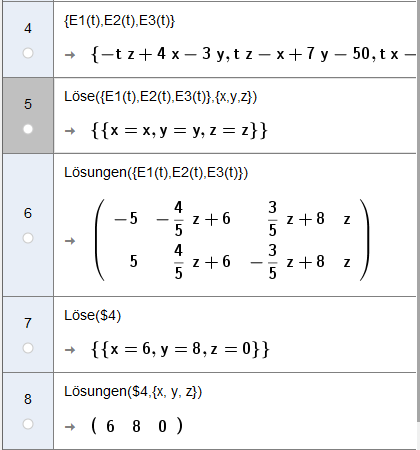# CAS: Different solve results

hawe shared this problem 1 year ago
New

how would you like to have it:

E1(k) = -k z + 4x - 3y

E2(k) = k z - x + 7y - 50

E3(k) = k x - 6k - 4z1

x, y, z are special so

```Solve(({-t z + 4x - 3y, t z - x + 7y - 50, t x - 6t - 4z}))
```

is the same as

```Solve(({-t z + 4x - 3y, t z - x + 7y - 50, t x - 6t - 4z}),{x,y,z})
```

Try

`Solve(({-t zz + 4xx - 3yy, t zz - xx + 7yy - 50, t xx - 6t - 4zz}))`